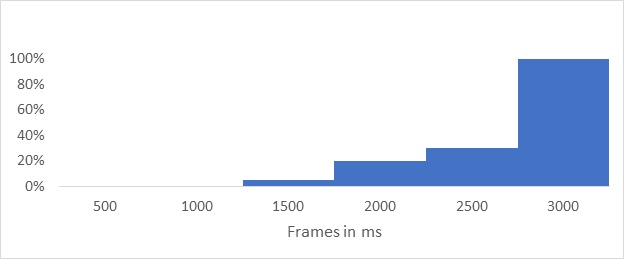Back to learning

Speed Index is a web performance metric invented by WebPageTest developers in 2012, and measures how quickly visual content is displayed as a website page loads. As speed index is actually measuring what an end user sees in their browser, it is a much better indicator of user perceived performance that metrics like the dom load or page load event.

#### How is it calculated?

As the website being measured is loaded, a series of screen shots are taken as content is loaded. Each screen shot is then scored for visual completeness 'above the fold' using the following formula:

``````Interval time * (1 - visual completeness/100)
``````

The score for each frame is calculated until all content is displayed and then all the scores are added to give you the speed index, the lower the score the better.

Here's an example with a 500ms interval:1 500 * (1 - 0/100) = 500 2 500 * (1 - 0/100) = 500 3 500 * (1 - 5/100) = 475 4 500 * (1 - 20/100) = 400 5 500 * (1 - 30/100) = 350 6 500 * (1 - 100/100) = 0

``````500 + 500 + 475 + 400 + 350 + 0 = 2225